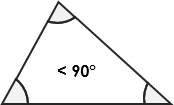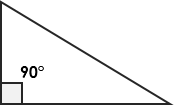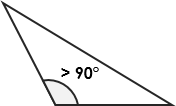# Triangle Area Calculator

If you’re wondering how to calculate the area of a triangle, this area of a triangle calculator will solve your problem in a blink of an eye! All you have to do is input the base and height of your triangle, and allow this triangle calculator to show you the answer in an instant. Keep reading if you want to understand the triangle area formula or if you want to learn how to find the area of a triangle.

## What is a triangle?

A triangle is a polygon that has three vertices that are joined by three line segments called edges.

There are three types of triangles:All angles are less than 90°Has a right angle (90°)Has an angle larger than 90°

## Triangle area formula

The following is the calculation formula for the area of a triangle.

`area = b * h / 2`

where b and h are the base and height of the triangle.

The formula works for all types of triangles.

## How to find the area of a triangle?

You can use the triangle area calculator to find the area of a triangle:

1. Enter the length of the base of the triangle.
2. Enter the length of its height.
3. Our triangle calculator performs all the calculations for you and shows the result instantly.

#### This calculator is created with

the best online spreadsheet editor with excellent formula and editing capability.

For more calculators for finance, mathematics health, unit converters and more check out our calculators collection.